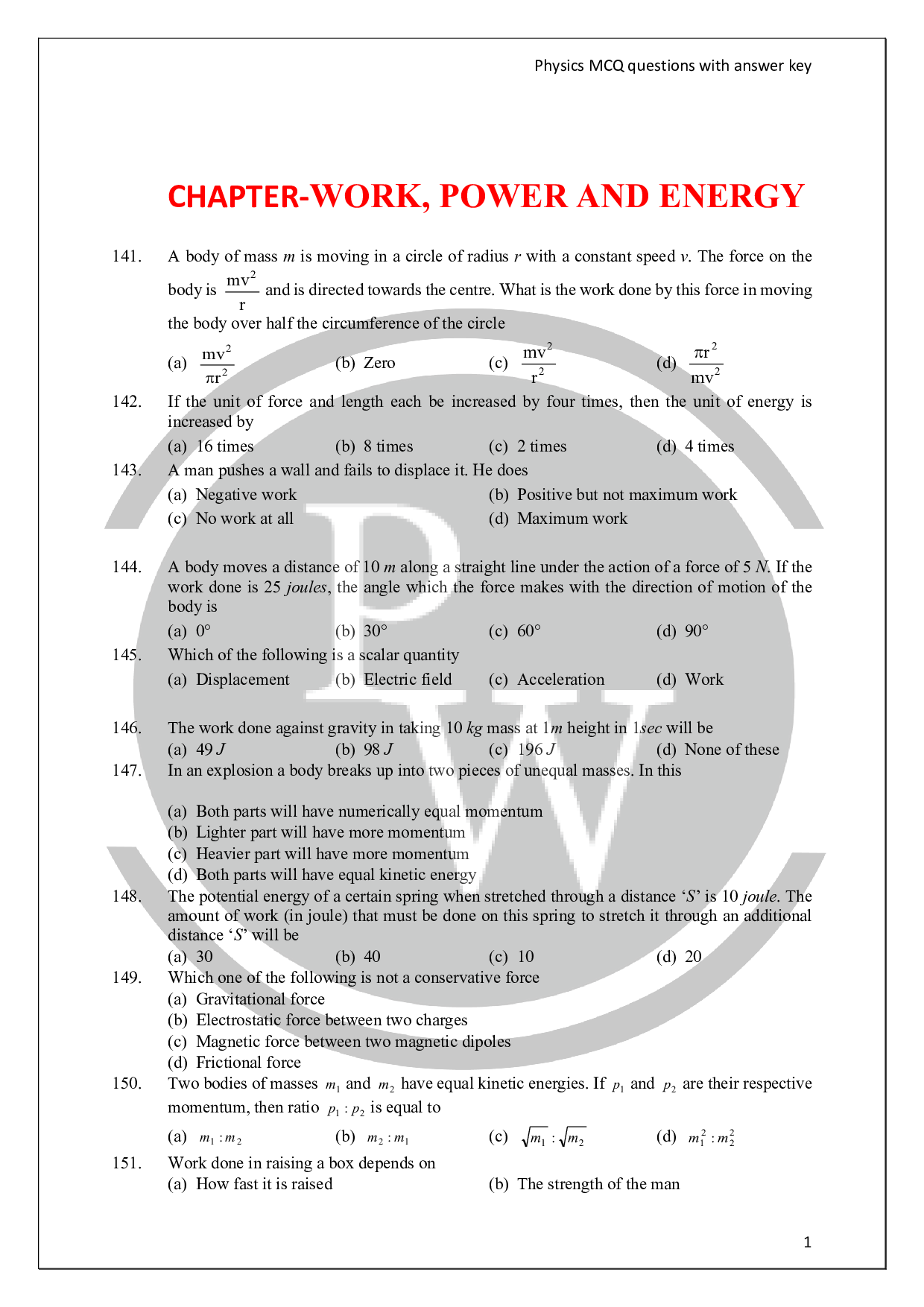# Work Energy And Power Questions

## About Work energy and power questions

The concept of work-energy is one of the most important concepts in science. It is an important concept that we use in physics, engineering chemistry, biology, geology and astronomy. In this lesson, we shall concentrate over mechanics related situations only. We shall first introduce the concept of work, and then energy and finally we shall see the concept of work-energy theorem. We shall see that the concept of work and energy can be applied to the dynamics of mechanical system without resorting to Newton’s laws.‘Energy approach’ often provides much simpler analysis than the direct applications of Newton’s laws in complex situations.Do check out chapter wise Physics Questions prepared by Entrancei.

### Find below pdf of Work Energy And Power Questions